## Definition

A$L^1$-regularized quadratic function of one variable is a function of the form: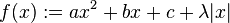$f(x) := ax^2 + bx + c + \lambda|x|$

where$a,b,c,\lambda \in \R, a > 0, \lambda > 0$. Note that we assume$a > 0$ for some of our case analysis, because we consider such functions in the context of modifying the minimization problem for quadratic functions.

The function has a piecewise quadratic function definition: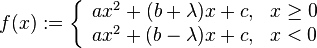$f(x) := \left\lbrace \begin{array}{rl} ax^2 + (b + \lambda)x + c, & x \ge 0 \\ ax^2 + (b - \lambda)x + c, & x < 0 \\\end{array}\right.$

## Differentiation

### First derivative

The expression for the first derivative is obtained from the piecewise definition of the function, using the differentiation rule for piecewise definition by interval. We obtain the expression: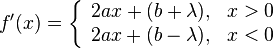$f'(x) = \left\lbrace \begin{array}{rl} 2ax + (b + \lambda), & x > 0 \\ 2ax + (b - \lambda), & x < 0 \\\end{array}\right.$

The first derivative is undefined at$x = 0$. At this point, the left-hand derivative is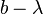$b - \lambda$ and the right-hand derivative is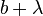$b + \lambda$.

The derivative function is therefore a piecewise linear function with a jump discontinuity at zero.

### Second derivative

The second derivative is defined as:$f''(x) = 2a, \qquad x \ne 0$

The second derivative is undefined at zero.

## Points and intervals of interest

### Critical points

As noted above, we have the following expression for the derivative:$f'(x) = \left\lbrace \begin{array}{rl} 2ax + (b + \lambda), & x > 0 \\ 2ax + (b - \lambda), & x < 0 \\\end{array}\right.$

We have at least one and at most three critical points. The three candidates are discussed below.

Critical point Case that it occurs Description of critical point
0 Always The function is not differentiable at the point. The left-hand derivative is$b - \lambda$ and the right-hand derivative is$b + \lambda$.$\frac{-(b + \lambda)}{2a}$ The case that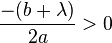$\frac{-(b + \lambda)}{2a} > 0$, or equivalently,$b < -\lambda$ It is the unique critical point for the quadratic piece for$x > 0$. It occurs if and only if the critical point for that piece happens to fall within that piece definition.$\frac{-(b - \lambda)}{2a}$ The case that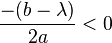$\frac{-(b - \lambda)}{2a} < 0$, or equivalently,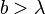$b > \lambda$ It is the unique critical point for the quadratic piece for$x < 0$. It occurs if and only if the critical point for that piece happens to fall within that piece definition.

The second and third critical point type cannot both occur. This can be seen arithmetically, since subtracting the second condition from the first contradicts the assumption pair$\lambda > 0, a > 0$. It also follows from the fact that the graph of the function is concave up (more on this later).

Here are the combined cases:

Case Set of critical points$0 > \frac{-(b - \lambda)}{2a}$, or equivalently,$b > \lambda$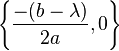$\left \{\frac{-(b - \lambda)}{2a}, 0 \right\}$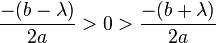$\frac{-(b - \lambda)}{2a} > 0 > \frac{-(b + \lambda)}{2a}$, or equivalently,$|b| < \lambda$$\{0 \}$$\frac{-(b + \lambda)}{2a} > 0$, or equivalently,$b < -\lambda$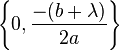$\left \{ 0, \frac{-(b + \lambda)}{2a} \right \}$

### Intervals of increase and decrease

We include the three cases below. Recall the assumption that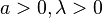$a > 0, \lambda > 0$:

Case Set of critical points$0 > \frac{-(b - \lambda)}{2a}$, or equivalently,$b > \lambda$ decreasing on$\left(-\infty,\frac{-(b - \lambda)}{2a}\right]$
increasing on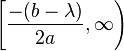$\left[\frac{-(b - \lambda)}{2a},\infty\right)$: note that the derivative is undefined at 0, but the function is continuous and increasing on both the left and right.$\frac{-(b - \lambda)}{2a} > 0 > \frac{-(b + \lambda)}{2a}$, or equivalently,$|b| < \lambda$ decreasing on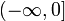$(-\infty,0]$.
increasing on$[0,\infty)$.$\frac{-(b + \lambda)}{2a} > 0$, or equivalently,$b < -\lambda$ decreasing on$\left(-\infty,\frac{-(b + \lambda)}{2a}\right]$: note that the derivative is undefined at 0, but the function is continuous and increasing on both the left and right.
increasing on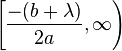$\left[\frac{-(b + \lambda)}{2a},\infty\right)$

### Local extreme values

We include the three cases below. Recall the assumption that$a > 0, \lambda > 0$. Note that the function is concave up (i.e., a convex function) and therefore has a unique point of local minimum that is also a point of absolute minimum.

Case Point of unique local minimum that is also a point of absolute minimum (value of$x$) Distance from the point of unique local minimum in the case$\lambda = 0$, namely, the value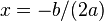$x = -b/(2a)$ Local minimum value (also the absolute minimum value) Distance from the local minimum value in the case$\lambda = 0$, namely, the value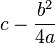$c - \frac{b^2}{4a}$ Extent to which the value at the point$x = -b/(2a)$ differs from the local minimum value (for the regularized function)$0 > \frac{-(b - \lambda)}{2a}$, or equivalently,$b > \lambda$$\frac{-(b - \lambda)}{2a}$$\frac{\lambda}{2a}$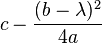$c - \frac{(b - \lambda)^2}{4a}$$\frac{\lambda(2b - \lambda)}{4a}$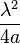$\frac{\lambda^2}{4a}$$\frac{-(b - \lambda)}{2a} > 0 > \frac{-(b + \lambda)}{2a}$, or equivalently,$|b| < \lambda$ 0$\frac{|b|}{2a}$$c$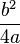$\frac{b^2}{4a}$$\frac{|b|(2\lambda - |b|)}{4a}$$\frac{-(b + \lambda)}{2a} > 0$, or equivalently,$b < -\lambda$$\frac{-(b + \lambda)}{2a}$$\frac{\lambda}{2a}$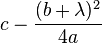$c - \frac{(b + \lambda)^2}{4a}$$\frac{\lambda(2b + \lambda)}{4a}$$\frac{\lambda^2}{4a}$

#### Single expression

The following single expression can be used to determine the point of local minimum: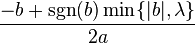$\frac{-b + \operatorname{sgn}(b)\min \{|b|,\lambda \} }{2a}$

Here is the intuitive explanation: we are starting out with the point of local minimum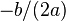$-b/(2a)$ for the original function. We then need to "move" a distance$\lambda/(2a)$ reflecting a change in the coefficient of$x$ by$\lambda$. The direction of move depends on the sign of$b$, because that affects what piece definition we are currently in. However, when moving, we do not want to cross the origin, because if the graph shifted that much, we lost the interior minimum point completely and have to settle for the minimum at the point where the definition changes.

#### Sensitivity to regularization parameter

The derivative of the point of local minimum with respect to the parameter$\lambda$ is given as follows (the expression is obtained from the expression for the point of local minimum above):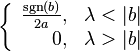$\left \lbrace \begin{array}{rl} \frac{\operatorname{sgn}(b)}{2a}, & \lambda < |b| \\ 0, & \lambda > |b| \\\end{array}\right.$

The derivative is undefined at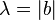$\lambda = |b|$. At this point, the point of local minimum transitions from a moving point to a stationary point at$x = 0$.

### Intervals of concave up and concave down

At all points other than 0, the function is twice differentiable and has positive second derivative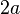$2a$. At zero, the first derivative jumps upward from$b - \lambda$ to$b + \lambda$. Therefore, the first derivative is increasing throughout the domain.